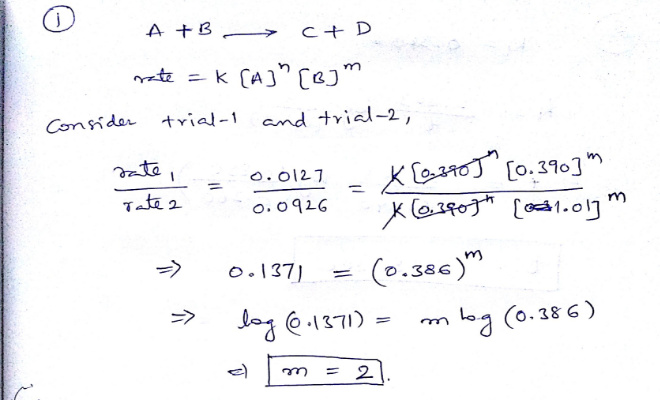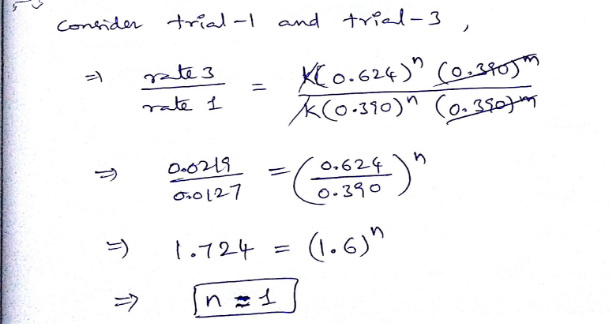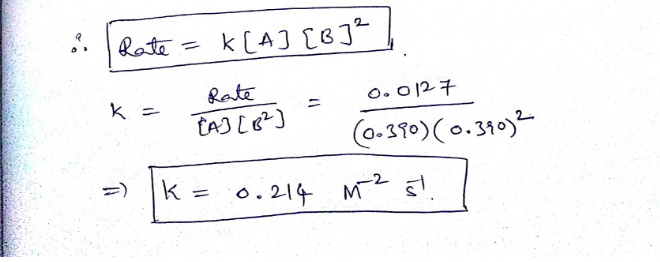# Using the given data, calculate the rate constant of this reaction

1.Using the given data, calculate the rate constant of this reaction.

A+B---------> C+D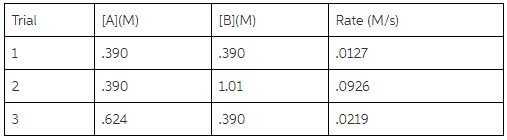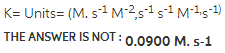2.The rate constant for this first-order reaction is 0.340 s–1 at 400 °C.

A------> Products

How long (in seconds) would it take for the concentration of A to decrease from 0.850 M to 0.260 M?